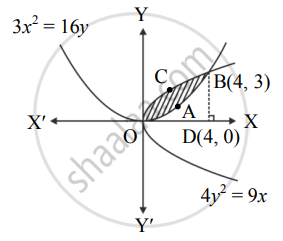# Find the area of the region lying between the parabolas 4y2 = 9x and 3x2 = 16y - Mathematics and Statistics

Sum

Find the area of the region lying between the parabolas 4y2 = 9x and 3x2 = 16y

#### Solution

Given equations of the parabolas are

4y2 = 9x       .......(i)

and 3x2 = 16y

∴ y = (3x^2)/16    ......(ii)

From (i), we get

y2 = 9/4x

∴ y = 3/2 sqrt(x)  ....(iii)  ......[∵ In first quadrant, y > 0]

Find the points of intersection of 4y2 = 9x and 3x2 = 16y.

Substituting (ii) in (i), we get

4((3x^2)/16)^2 = 9x

∴ x4 = 64x

∴ x4 – 64x = 0

∴ x(x3 – 64) = 0

∴ x = 0 or x3 = 64 = 43

∴ x = 0 or x = 4

When x = 0, y = 0 and when x = 4, y = 3

∴ The points of intersection are O(0, 0) and B(4, 3).

Draw BD ⊥ OX.Required area = area of the region OABCO

= area of the region ODBCO – area of the region ODBAO

= area under the parabola 4y2 = 9x – area under the parabola 3x2 = 16y

= int_0^4 3/2 sqrt(x)  "d"x - int_0^4  (3x^2)/16  "d"x

= 3/2 int_0^4 x^(1/2)  "d"x - 3/16  int_0^4  x^2  "d"x

= 3/2[(x^(3/2))/(3/2)]_0^4 - 3/16[x^3/3]_0^4

= [(4)^(3/2) - 0] - 1/16[(4)^3 - 0]

= 8 - 1/16 (64)

= 8 – 4

= 4 sq.units

Concept: Area Between Two Curves
Is there an error in this question or solution?